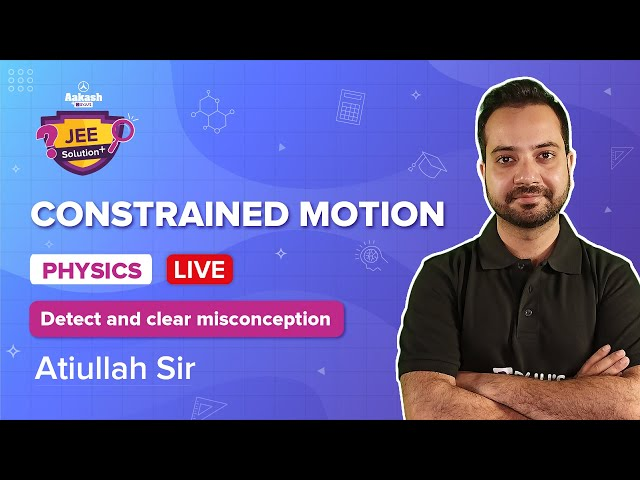# Laws of Motion- Important Questions in JEE Main

Laws of Motion is one of the major topics in physics as it lays the foundation for the entire mechanics. Let’s take a look at a few FAQs related to the topic.

## Important Questions For Laws Of Motion

1. What are the major concepts involved in the topic?

The major concepts involve Frame of reference, Newton’s First law of motion (Law of Inertia), Newton’s Second law of motion, Newton’s Third law of motion (For every action there is an equal and opposite reaction) and constraint equations.

2. What are the characteristics of Normal Force?

The normal force is an electromagnetic force and always acts perpendicular to the surface of contact.

3. What do we understand by Free Body Diagram?

A free body diagram represents all the forces acting on the body. For example,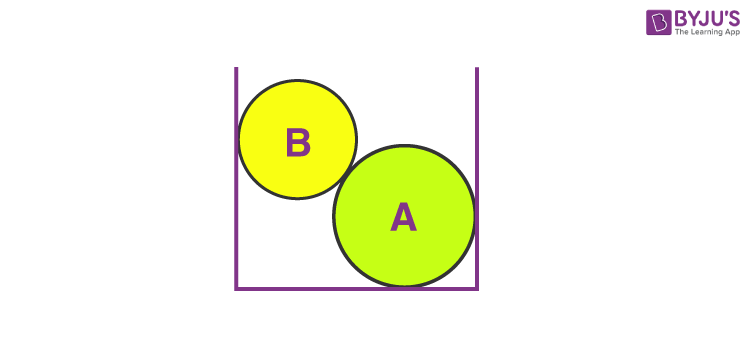So, the FBD for sphere B will be as shown below,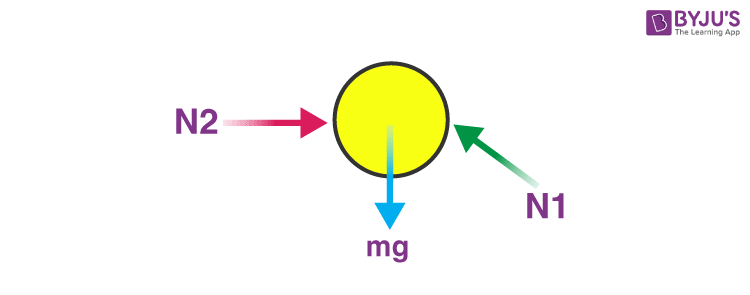4. What are the steps to solve a problem on Newton’s laws of motion?

Let’s take the following example,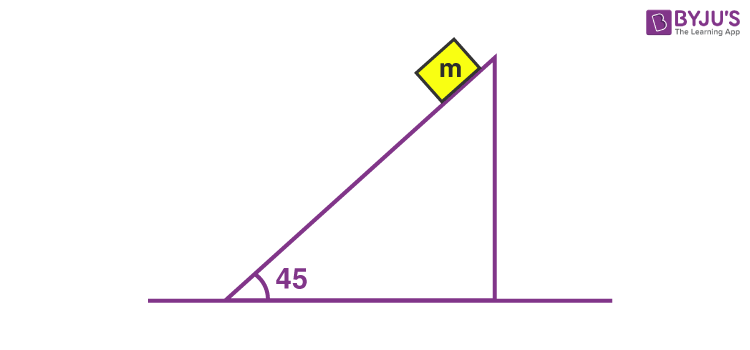The wedge is fixed and we need to find the acceleration of the block of mass m along the incline.

Step 1: Draw the F.B.D of the block,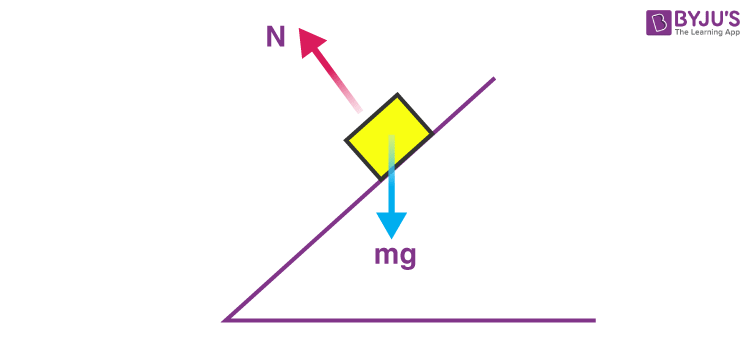Step 2: Writing the force equation taking our own axis, in this case, we take two axes one along the plane and the other perpendicular to it.

FIncline = mg sin 45o

VFNormal = N – mg cos 45o

Step3: Calculating acceleration using the second law of motion.

mg sin 45o = ma

•  a = g sin 45o

5. What is the Constraint Equation?

When the motion of one body is governed by another or in other words when the motion of two or more bodies is interlinked. The equation governing such motion is known as the constraint equation. For example: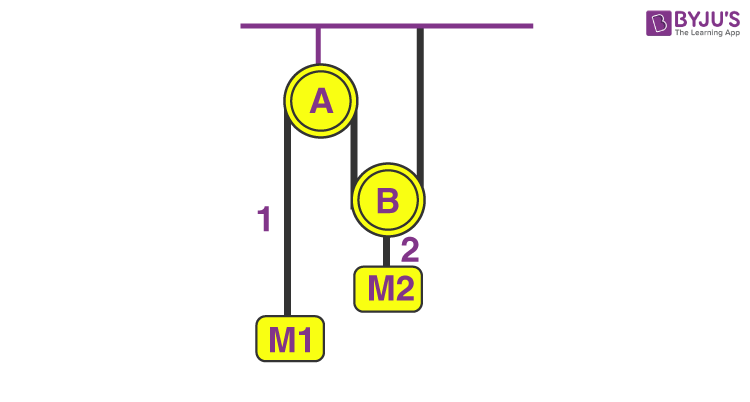If we draw the FBD and write equations we will get,

M1.g – T = M1.a1

T – M2.g = M2.a2

We can see there are two equations and three unknowns: a1, a2 and T. So we need one more equation and that will be the constraint equation.

To know more about Newton’s Laws of motion get in touch with our mentors at BYJU’S.

#### Also Watch – Contrained Motion JEE Solution Video